Mode elimination: taking the phases into account: 3

In this post we look at some of the fundamental problems involved in taking a conditional average over the high-wavenumber modes, while leaving the low-wavenumber modes unaffected.

Let us consider isotropic, stationary turbulence, with a velocity field in wavenumber space which is defined on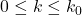. Note that the maximum wavenumber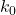is not the Kolmogorov dissipation wavenumber, although in both large-eddy simulation and in the application of renormalisation group (RG) to turbulence, it is often taken to be so. The only definition that I know of, is the one I put forward in 1986 , which is:

(1)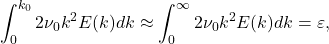where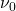is the kinematic viscosity of the fluid,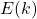is the energy spectrum, and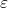is the dissipation rate. Obviously the value ofdepends on how closely the integral on the left approximates the actual dissipation rate, which corresponds to the upper limit on the integral being taken as infinity. Some people apparently find this definition puzzling, possibly because they are familiar with RG in the context of the theory of critical phenomena, where the maximum wavenumber is determined by the inverse of the lattice constant. In contrast, fluid dynamicists may find our definition here quite intuitive, as it is analogous to Prandtl’s definition of the laminar boundary layer.

Something which may be counter-intuitive for many, is the choice ofas the maximum wavenumber. This is because in RG we progressively eliminate modes in wavenumber bands: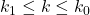,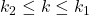,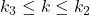, and so on, where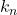decreases with increasing integer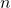, until the iteration reaches a fixed point. Also, the fluid viscosityis so denoted, because it is progressively renormalized until it reaches a value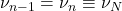, at the fixed point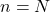.

The first step in eliminating a band of modes is quite straightforward. We high-pass, and low-pass, filter the velocity field at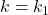, thus:

(2)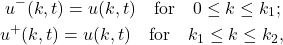where we have adopted a simplified notation. Then we can substitute the decomposition given by equation (2) into the Navier-Stokes equation in wavenumber, and study the effect. However we will not pursue that here, and further details can be found in Section 5.1.1 of . Instead, we will concentrate here on the following question: how do we average out the effect of the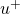modes, while keeping the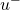modes constant?

The condition for such an average can be written as:

(3)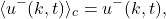where the subscript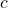‘ denotes conditional’. We should also recall that isotropic turbulence requires a zero mean velocity, that is: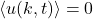.

Actually, it would be quite simple to carry out such an average, provided that the velocity field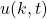were multivariate normal. In that case, each of the various modes could be averaged out, independently of all the rest. However, the turbulent velocity field is not Gaussian so, in attempting to carry out a such an average, we would run into the following two problems.

First, we must satisfy the boundary condition between the two regions of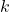-space. Hence,

(4)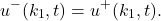This is the extreme case, where we would be trying to average out a high-mode while leaving the identical low-mode unaffected. At the very least, this draws attention to the need for scale separation.

Secondly, there are some questions about the nature of the averaging over modes, in terms of the averaging of the velocity field in real space. In order to consider this, let us introduce a combined Fourier transform and filter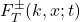acting on the velocity field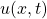, such that:

(5)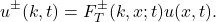Noting that both the Fourier transform and the filter are purely deterministic entities, the average can only act on the real-space velocity field, leading to zero!

So it seems that a simple filtered average, as used in various attempts at subgrid modelling or RG applied to turbulence, cannot be correct at a fundamental level. We will see in the next post how the introduction of a particular kind of conditional average led to a more satisfactory situation .

 W. D. McComb. Application of Renormalization Group methods to the subgrid modelling problem. In U. Schumann and R. Friedrich, editors, Direct and Large Eddy Simulation of Turbulence, pages 67- 81. Vieweg, 1986.
 W. David McComb. Homogeneous, Isotropic Turbulence: Phenomenology, Renormalization and Statistical Closures. Oxford University Press, 2014.
 W. D. McComb, W. Roberts, and A. G. Watt. Conditional-averaging procedure for problems with mode-mode coupling. Phys. Rev. A, 45(6):3507- 3515, 1992.